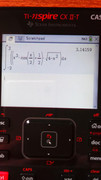HP Forums

Full Version: Attached integral calculator cannot solve
You're currently viewing a stripped down version of our content. View the full version with proper formatting.
Attached integral calculator cannot solve
Have you tried numerical integration? No Prime here, but on the HP 50g we can do

'∫(-2,2,(X^3*COS(X/2)+1/2)*√(4-X^2),X)'

→NUM

3.14159265359
CX II yields the same result (that is pi), try numerical mode as mentionedYou just have to realise that

'∫(-2,2,(X^3*COS(X/2)+1/2)*√(4-X^2),X)'
= '∫(-2,2,X^3*COS(X/2)*√(4-X^2),X)' + '∫(-2,2,1/2*√(4-X^2),X)'

The first part is an odd function, so the integral is zero, and we are left with the easy second part.

Cheers, Werner
(11-15-2019 11:15 AM)yingwuluo Wrote: [ -> ]Attached integral calculator cannot solve

numerically: int((x^3*cos(x/2)+1/2)*√(4-X^2),x,-2,2) [shift] [enter] returns 3.14159265359.

symbolically: simplify(subst(int((x^3*cos(x/2)+1/2)*√(4-X^2),x,-2,2), x=2*sin(t))) returns pi.
With that answer, to access the WiFi network, do you suppose the 10th digit should be a 3 or a 4 ? Setting a calculator to Fix 9 would lead most folks to use the 4, while the 10th digit actually is 3. Not sure what the network admin had in mind. Who would guess that numerical rounding rules would need to be specified for a WiFi password?(11-15-2019 11:15 AM)yingwuluo Wrote: [ -> ]Attached integral calculator cannot solve

It works for me:(11-15-2019 12:56 PM)Werner Wrote: [ -> ]You just have to realise that

'∫(-2,2,(X^3*COS(X/2)+1/2)*√(4-X^2),X)'
= '∫(-2,2,X^3*COS(X/2)*√(4-X^2),X)' + '∫(-2,2,1/2*√(4-X^2),X)'

The first part is an odd function, so the integral is zero, and we are left with the easy second part.

To finish, second part = ½ area of half-circle (radius 2) = ½ (2 pi) = pi

(11-15-2019 02:04 PM)rprosperi Wrote: [ -> ]With that answer, to access the WiFi network, do you suppose the 10th digit should be a 3 or a 4 ?

That implied round-toward-zero ⇒ password = 3141592653(11-15-2019 02:25 PM)Albert Chan Wrote: [ -> ]
(11-15-2019 12:56 PM)Werner Wrote: [ -> ]You just have to realise that

'∫(-2,2,(X^3*COS(X/2)+1/2)*√(4-X^2),X)'
= '∫(-2,2,X^3*COS(X/2)*√(4-X^2),X)' + '∫(-2,2,1/2*√(4-X^2),X)'

The first part is an odd function, so the integral is zero, and we are left with the easy second part.

To finish, second part = ½ area of half-circle (radius 2) = ½ (2 pi) = pi

(11-15-2019 02:04 PM)rprosperi Wrote: [ -> ]With that answer, to access the WiFi network, do you suppose the 10th digit should be a 3 or a 4 ?

That implied round-toward-zero ⇒ password = 3141592653Statisticians either round half to even or round half to odd:

Round half to even:
1.235 ==> 1.24
1.245 ==> 1.24
1.255 ==> 1.26
1.265 ==> 1.26

Round half to odd:
1.225 ==> 1.23
1.235 ==> 1.23
1.245 ==> 1.25
1.255 ==> 1.25

This way the rounding error is minimized in large populations of data.
Just for the fun of it, I tried the definite integral on my CASIO fix-991EX, and it came up with the symbol for pi!
(11-15-2019 07:02 PM)lrdheat Wrote: [ -> ]Just for the fun of it, I tried the definite integral on my CASIO fix-991EX, and it came up with the symbol for pi!

Well... that's only 2 digits! No WiFi for you!
Seinfeld! Quotable for almost every aspect of life!
Using Mathematica Home Edition 12.0, it took a little longer than usual with other equations but finally produced the Pi symbol. On my G2 it returned the equation unsolved, but when I hit Simplify, it returned Pi.
The Prime G1 had no problem coming up with the Pi symbol in CAS or 3.141592... in Home right away. Odd thing was both worked straight off using lowercase x, then when I tried later in Home received an "Error: Syntax Error" message until I changed to an uppercase X. Took 1.034_s in CAS and .588_s in Home.

BTW, also tried solving in Mathematica 12 Home Edition on an 8-core, 64 GB RAM PC with 2 TB SSD. It took .656 seconds to return the Pi symbol, slightly longer than the HP Prime G1 in Home mode.
Reference URL's
• HP Forums: https://www.hpmuseum.org/forum/index.php
• :